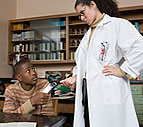×

# multiply

1 ENTRIES FOUND:
multiply /ˈmʌltəˌplaɪ/ verb
multiplies; multiplied; multiplying
multiply
/ˈmʌltəˌplaɪ/
verb
multiplies; multiplied; multiplying
Learner's definition of MULTIPLY
[no object] : to increase greatly in number or amount : to become much more numerous
[+ object] : to cause (something) to increase greatly in number or amount
[no object] : to increase in number by reproducing
mathematics : to add a number to itself a certain number of times
[+ object]
[no object]
compare divide